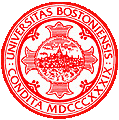# Homework 1: Programming Assignment

CS 585 HW 1
Shunying Chen
1/28/2020

## Problem Definition

The problem or purpose of this assignment is mainly to explore the features of OpenCV using Visual Studio. We need to utilize these two resources to manipulate our self-portraits in 3 ways:

1. Create a greyscale version of our portrait.
2. Flip our portrait horizontally, i.e. left to right or right to left.
3. Anything we would like, but I manipulated it by blurring the image.

This assignment is useful in understanding the features we could use with OpenCV to later on create much more complicated projects, and solve future coding assignments. I am assuming that I can use any provided OpenCV functions to solve some of the problems rather than writing a full function. The anticipated difficulties will be knowing the syntax and functions I should use to manipulate the image.

## Method and Implementation

Brief description of the image manipulations:

1. The greyscale image was manipulated by taking in the original image, and for each pixel the RGB values are averaged, and replaced by the averaged value. I used this version instead of weighting the RGB values instead just for the simplicity. The function will be listed below.
2. The flipped image was created by swapping the RGB values of each pixel with its corresponding mirror pixel therefore resulting in a flipped image. The function will be listed below.
3. The blurred image was created by taking in the greyscale image, and for each pixel the average is taken of the pixel itself and its surrounding pixels. Once the value is calculated, the RGB values are replaced by the value. There are special cases when calculating the values such as the corners and the edges, since there is not 8 pixels surrounding it. I blurred the image 5 times to make it really noticable. This was done with a for loop inside the blur function. The function will be listed below.

Functions:

void turnGrey(Mat& image); void flipH(Mat& image); void blur(Mat& greyimage);

## Experiments

My main function reads the inputted image of me, and for each manipulation a clone of the original image was made and passed into each function. Then all the original and manipulated images are displayed in a window and to see if they are what they are supposed to look like.

## Results

### Results

Trial Source Image Result Image
GreyFlipBlur